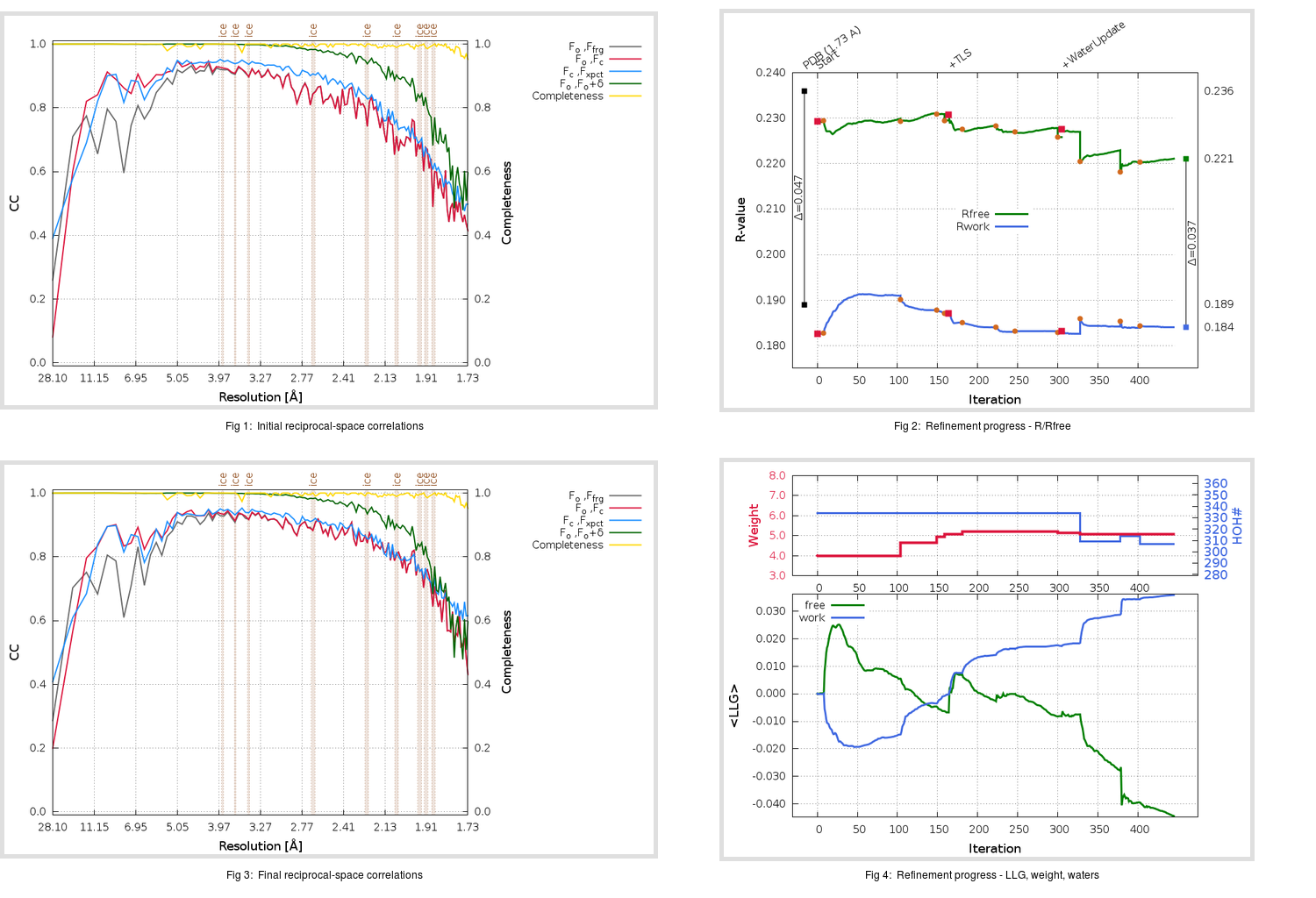Content:

```    Diffraction limits & principal axes of ellipsoid fitted to diffraction cut-off surface:
1.917         0.9839   0.0000  -0.1786       0.988 a* - 0.155 c*
1.749         0.0000   1.0000   0.0000       b*
1.654         0.1786   0.0000   0.9839       0.441 a* + 0.898 c*
```

## Deposited

` `
 Date deposited Date data collection Resolution R, Rfree 20200315 20200305 1.73 0.1860 0.2360

Molprobity (CCP4 7.0 version) summary:

```Ramachandran outliers =   0.33 %
favored =  97.02 %
Rotamer outliers      =   1.14 %
C-beta deviations     =     0
Clashscore            =   4.85
RMS(bonds)            =   0.0133
RMS(angles)           =   1.82
MolProbity score      =   1.47
Resolution            =   1.73
R-work                =   0.1860
R-free                =   0.2360
```

```Number of waters      =   334

<B> (all atoms) =   28.22 ( sd =   10.18 ) for       2731 non-hydrogen atoms
<B>   (protein) =   26.60 ( sd =    9.16 ) for       2370 non-hydrogen atoms
<B>     (water) =   38.95 ( sd =   10.36 ) for        334 non-hydrogen atoms
<B>    (others) =   37.53 ( sd =   10.75 ) for         27 non-hydrogen atoms

B min/max       (all non-hydrogen atoms) =   13.84 /   81.18
B min/max   (protein non-hydrogen atoms) =   14.52 /   81.18
B min/max     (water non-hydrogen atoms) =   13.84 /   75.06
B min/max     (other non-hydrogen atoms) =   32.13 /   51.07
```

## BUSTER (re-)refinement

` `

Molprobity (CCP4 7.0 version) summary:

```Ramachandran outliers =   0.33 %
favored =  97.68 %
Rotamer outliers      =   1.52 %
C-beta deviations     =     0
Clashscore            =   4.01
RMS(bonds)            =   0.0118
RMS(angles)           =   1.57
MolProbity score      =   1.39
Resolution            =   1.73
R-work                =   0.1841
R-free                =   0.2211
```

```Number of waters      =   307

<B> (all atoms) =   29.41 ( sd =    9.04 ) for       2704 non-hydrogen atoms
<B>   (protein) =   27.68 ( sd =    7.31 ) for       2370 non-hydrogen atoms
<B>     (water) =   41.72 ( sd =   10.70 ) for        307 non-hydrogen atoms
<B>    (others) =   41.91 ( sd =   12.04 ) for         27 non-hydrogen atoms

B min/max       (all non-hydrogen atoms) =   15.19 /   88.01
B min/max   (protein non-hydrogen atoms) =   15.19 /   59.95
B min/max     (water non-hydrogen atoms) =   17.73 /   88.01
B min/max     (other non-hydrogen atoms) =   34.74 /   54.89
```

Refinement progression:Results:

` `
 File Remark 5RF1_aB_refine.01_03_refine.pdb.gz exact refinement commands are in header 5RF1_aB_refine.01_03_refine.mtz.gz including original deposited data and several re-refinement map coefficients 5RF1_aB_refine.01_03_BUSTER_model.cif.gz including any non-standard compound restraints 5RF1_aB_refine.01_03_BUSTER_refln.cif.gz